# 7.7 Graphing linear inequalities in two variables

 Page 1 / 1
This module is from Elementary Algebra by Denny Burzynski and Wade Ellis, Jr. In this chapter the student is shown how graphs provide information that is not always evident from the equation alone. The chapter begins by establishing the relationship between the variables in an equation, the number of coordinate axes necessary to construct its graph, and the spatial dimension of both the coordinate system and the graph. Interpretation of graphs is also emphasized throughout the chapter, beginning with the plotting of points. The slope formula is fully developed, progressing from verbal phrases to mathematical expressions. The expressions are then formed into an equation by explicitly stating that a ratio is a comparison of two quantities of the same type (e.g., distance, weight, or money). This approach benefits students who take future courses that use graphs to display information.The student is shown how to graph lines using the intercept method, the table method, and the slope-intercept method, as well as how to distinguish, by inspection, oblique and horizontal/vertical lines. Objectives of this module: be able to locate solutions to linear inequalities in two variables using graphical techniques.

## Overview

• Location of Solutions
• Method of Graphing

## Location of solutions

In our study of linear equations in two variables, we observed that all the solutions to the equation, and only the solutions to the equation, were located on the graph of the equation. We now wish to determine the location of the solutions to linear inequalities in two variables. Linear inequalities in two variables are inequalities of the forms:

$\begin{array}{cc}ax+by\le c& ax+by\ge c\\ ax+byc\end{array}$

## Half-planes

A straight line drawn through the plane divides the plane into two half-planes .

## Boundary line

The straight line is called the boundary line .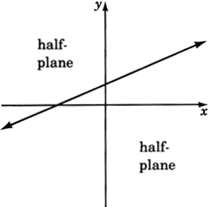## Solution to an inequality in two variables

Recall that when working with linear equations in two variables, we observed that ordered pairs that produced true statements when substituted into an equation were called solutions to that equation. We can make a similar statement for inequalities in two variables. We say that an inequality in two variables has a solution when a pair of values has been found such that when these values are substituted into the inequality a true statement results.

## The location of solutions in the plane

As with equations, solutions to linear inequalities have particular locations in the plane. All solutions to a linear inequality in two variables are located in one and only in one entire half-plane. For example, consider the inequality

$2x+3y\le 6$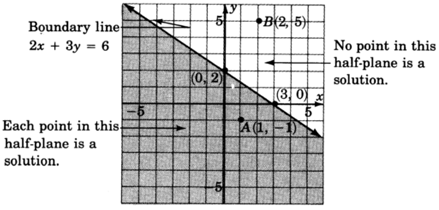All the solutions to the inequality $2x+3y\le 6$ lie in the shaded half-plane.

Point $A\left(1,\text{\hspace{0.17em}}-1\right)$ is a solution since

$\begin{array}{l}2x+3y\le 6\hfill \\ 2\left(1\right)+3\left(-1\right)\le 6?\hfill \\ 2-3\le 6?\hfill \\ -1\le 6.\text{\hspace{0.17em}}\text{\hspace{0.17em}}\text{\hspace{0.17em}}\text{\hspace{0.17em}}\text{\hspace{0.17em}}\text{True}\hfill \end{array}$

Point $B\left(2,\text{\hspace{0.17em}}5\right)$ is not a solution since

$\begin{array}{l}2x+3y\le 6\hfill \\ 2\left(2\right)+3\left(5\right)\le 6?\hfill \\ 4+15\le 6?\hfill \\ 19\le 6.\text{\hspace{0.17em}}\text{\hspace{0.17em}}\text{\hspace{0.17em}}\text{\hspace{0.17em}}\text{\hspace{0.17em}}\text{\hspace{0.17em}}\text{\hspace{0.17em}}\text{\hspace{0.17em}}\text{\hspace{0.17em}}\text{\hspace{0.17em}}\mathrm{False}\hfill \end{array}$

## Method of graphing

The method of graphing linear inequalities in two variables is as follows:

1. Graph the boundary line (consider the inequality as an equation, that is, replace the inequality sign with an equal sign).
1. If the inequality is $\le$ or $\ge$ , draw the boundary line solid . This means that points on the line are solutions and are part of the graph.
2. If the inequality is $<$ or $>$ , draw the boundary line dotted . This means that points on the line are not solutions and are not part of the graph.
2. Determine which half-plane to shade by choosing a test point.
1. If, when substituted, the test point yields a true statement, shade the half-plane containing it.
2. If, when substituted, the test point yields a false statement, shade the half-plane on the opposite side of the boundary line.

## Sample set a

Graph $3x-2y\ge -\text{\hspace{0.17em}}4$ .

1. Graph the boundary line. The inequality is $\ge$ so we’ll draw the line solid . Consider the inequality as an equation.

$3x-2y=-4$

 $x$ $y$ $\left(x,\text{\hspace{0.17em}}y\right)$ $\begin{array}{r}\hfill 0\\ \hfill \frac{-4}{3}\end{array}$ $\begin{array}{c}2\\ \\ 0\end{array}$ $\begin{array}{l}\left(0,\text{\hspace{0.17em}}2\right)\hfill \\ \left(\frac{-4}{3},\text{\hspace{0.17em}}0\right)\hfill \end{array}$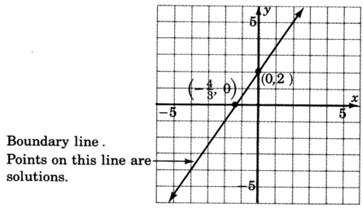2. Choose a test point. The easiest one is $\left(0,\text{\hspace{0.17em}}0\right)$ . Substitute $\left(0,\text{\hspace{0.17em}}0\right)$ into the original inequality.

$\begin{array}{l}3x-2y\ge -4\hfill \\ 3\left(0\right)-2\left(0\right)\ge -4?\hfill \\ 0-0\ge -4?\hfill \\ 0\ge -4.\text{\hspace{0.17em}}\text{\hspace{0.17em}}\text{\hspace{0.17em}}\text{\hspace{0.17em}}\text{\hspace{0.17em}}\text{\hspace{0.17em}}\text{\hspace{0.17em}}\text{\hspace{0.17em}}\text{\hspace{0.17em}}\text{\hspace{0.17em}}\mathrm{True}\hfill \end{array}$
Shade the half-plane containing $\left(0,\text{\hspace{0.17em}}0\right)$ .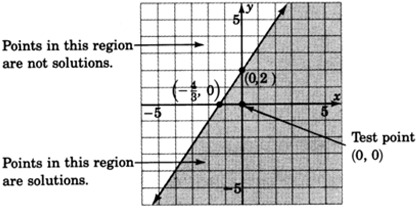Graph $x+y-3<0$ .

1. Graph the boundary line: $x+y-3=0$ . The inequality is $<$ so we’ll draw the line dotted .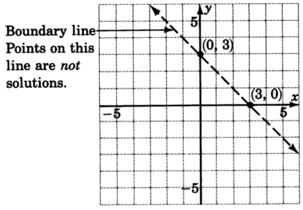2. Choose a test point, say $\left(0,\text{\hspace{0.17em}}0\right)$ .

$\begin{array}{l}x+y-3<0\hfill \\ 0+0-3<0?\hfill \\ -3<0.\text{\hspace{0.17em}}\text{\hspace{0.17em}}\text{\hspace{0.17em}}\text{\hspace{0.17em}}\text{\hspace{0.17em}}\text{\hspace{0.17em}}\text{\hspace{0.17em}}\text{\hspace{0.17em}}\mathrm{True}\hfill \end{array}$
Shade the half-plane containing $\left(0,\text{\hspace{0.17em}}0\right)$ .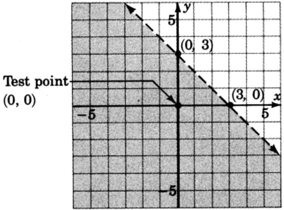Graph $y\le 2x$ .

1. Graph the boundary line $y=2x$ . The inequality is $\le$ , so we’ll draw the line solid .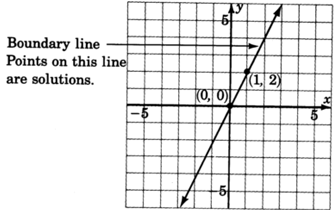2. Choose a test point, say $\left(0,\text{\hspace{0.17em}}0\right)$ .

$\begin{array}{l}y\le 2x\hfill \\ 0\le 2\left(0\right)?\hfill \\ 0\le 0.\text{\hspace{0.17em}}\text{\hspace{0.17em}}\text{\hspace{0.17em}}\text{\hspace{0.17em}}\text{\hspace{0.17em}}\text{\hspace{0.17em}}\mathrm{True}\hfill \end{array}$

Shade the half-plane containing $\left(0,\text{\hspace{0.17em}}0\right)$ . We can’t! $\left(0,\text{\hspace{0.17em}}0\right)$ is right on the line! Pick another test point, say $\left(1,\text{\hspace{0.17em}}6\right)$ .

$\begin{array}{l}y\le 2x\hfill \\ 6\le 2\left(1\right)?\hfill \\ 6\le 2.\text{\hspace{0.17em}}\text{\hspace{0.17em}}\text{\hspace{0.17em}}\text{\hspace{0.17em}}\text{\hspace{0.17em}}\text{\hspace{0.17em}}\text{\hspace{0.17em}}\mathrm{False}\hfill \end{array}$

Shade the half-plane on the opposite side of the boundary line.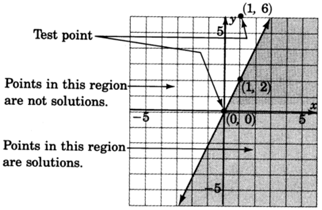Graph $y>2$ .

1. Graph the boundary line $y=2$ . The inequality is $>$ so we’ll draw the line dotted .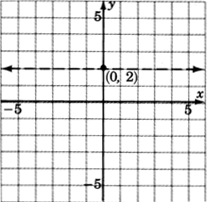2. We don’t really need a test point. Where is $y>2?$ Above the line $y=2!$ Any point above the line clearly has a $y\text{-coordinate}$ greater than 2.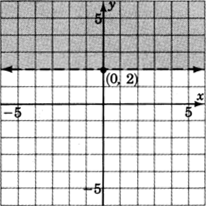## Practice set a

Solve the following inequalities by graphing.

$-3x+2y\le 4$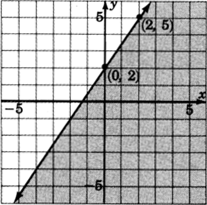$x-4y<4$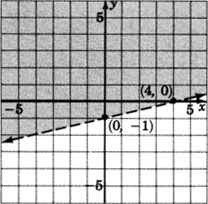$3x+y>0$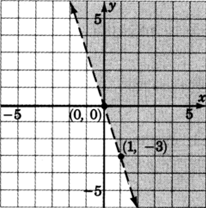$x\ge 1$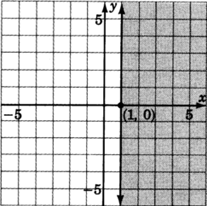## Exercises

Solve the inequalities by graphing.

$y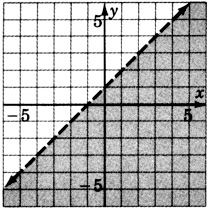$x+y\le 1$$-x+2y+4\ge 0$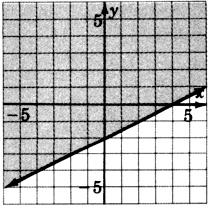$-x+5y-10<0$$-3x+4y>-12$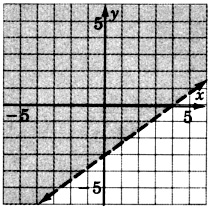$2x+5y-15\ge 0$$y\le 4$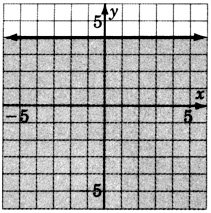$x\ge 2$$x\le 0$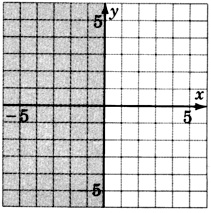$x-y<0$$x+3y\ge 0$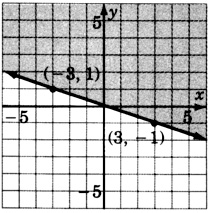$-2x+4y>0$## Exercises for review

( [link] ) Graph the inequality $-3x+5\ge -1$ .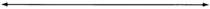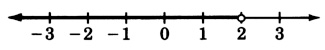( [link] ) Supply the missing word. The geometric representation (picture) of the solutions to an equation is called the of the equation.

( [link] ) Supply the denominator: $m=\frac{{y}_{2}-{y}_{1}}{?}$ .

$m=\frac{{y}_{2}-{y}_{1}}{{x}_{2}-{x}_{1}}$

( [link] ) Graph the equation $y=-3x+2$ .( [link] ) Write the equation of the line that has slope 4 and passes through the point $\left(-1,\text{\hspace{0.17em}}2\right)$ .

$y=4x+6$

where we get a research paper on Nano chemistry....?
nanopartical of organic/inorganic / physical chemistry , pdf / thesis / review
Ali
what are the products of Nano chemistry?
There are lots of products of nano chemistry... Like nano coatings.....carbon fiber.. And lots of others..
learn
Even nanotechnology is pretty much all about chemistry... Its the chemistry on quantum or atomic level
learn
da
no nanotechnology is also a part of physics and maths it requires angle formulas and some pressure regarding concepts
Bhagvanji
hey
Giriraj
Preparation and Applications of Nanomaterial for Drug Delivery
revolt
da
Application of nanotechnology in medicine
what is variations in raman spectra for nanomaterials
ya I also want to know the raman spectra
Bhagvanji
I only see partial conversation and what's the question here!
what about nanotechnology for water purification
please someone correct me if I'm wrong but I think one can use nanoparticles, specially silver nanoparticles for water treatment.
Damian
yes that's correct
Professor
I think
Professor
Nasa has use it in the 60's, copper as water purification in the moon travel.
Alexandre
nanocopper obvius
Alexandre
what is the stm
is there industrial application of fullrenes. What is the method to prepare fullrene on large scale.?
Rafiq
industrial application...? mmm I think on the medical side as drug carrier, but you should go deeper on your research, I may be wrong
Damian
How we are making nano material?
what is a peer
What is meant by 'nano scale'?
What is STMs full form?
LITNING
scanning tunneling microscope
Sahil
how nano science is used for hydrophobicity
Santosh
Do u think that Graphene and Fullrene fiber can be used to make Air Plane body structure the lightest and strongest. Rafiq
Rafiq
what is differents between GO and RGO?
Mahi
what is simplest way to understand the applications of nano robots used to detect the cancer affected cell of human body.? How this robot is carried to required site of body cell.? what will be the carrier material and how can be detected that correct delivery of drug is done Rafiq
Rafiq
if virus is killing to make ARTIFICIAL DNA OF GRAPHENE FOR KILLED THE VIRUS .THIS IS OUR ASSUMPTION
Anam
analytical skills graphene is prepared to kill any type viruses .
Anam
Any one who tell me about Preparation and application of Nanomaterial for drug Delivery
Hafiz
what is Nano technology ?
write examples of Nano molecule?
Bob
The nanotechnology is as new science, to scale nanometric
brayan
nanotechnology is the study, desing, synthesis, manipulation and application of materials and functional systems through control of matter at nanoscale
Damian
Is there any normative that regulates the use of silver nanoparticles?
what king of growth are you checking .?
Renato
What fields keep nano created devices from performing or assimulating ? Magnetic fields ? Are do they assimilate ?
why we need to study biomolecules, molecular biology in nanotechnology?
?
Kyle
yes I'm doing my masters in nanotechnology, we are being studying all these domains as well..
why?
what school?
Kyle
biomolecules are e building blocks of every organics and inorganic materials.
Joe
Please keep in mind that it's not allowed to promote any social groups (whatsapp, facebook, etc...), exchange phone numbers, email addresses or ask for personal information on QuizOver's platform.

#### Get Jobilize Job Search Mobile App in your pocket Now!ByByBy Nick SwainBy Jazzycazz JacksonBy Yasser IbrahimBy Ann SchlosserBy OpenStaxBy Stephen VoronBy Yasser IbrahimBy Michael NelsonByBy OpenStax